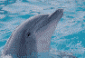# dimname problems

4 posts / 0 newOffline
Joined: 10/19/2009 - 15:38
dimname problems

there are inconsistencies in the documentation and execution of a model involving dimnames for matrices

i read in an observed cov matrix using read.table(..., header=TRUE, ...) and got this error in mxRun:
>Running thisOMxModel
>Error: The dataset associated with the ML objective in model 'MZ' does not contain identical row and column non-NULL dimnames.

fine. fixed that up, and the next mxRun gave this error:
>Running thisOMxModel
>Error: The expected covariance matrix associated with the ML objective in model 'MZ' does not contain dimnames.

the documentation does not mention that an MxData object of type='cov' (and possibly 'corr' and 'sscp') requires identical row and column names. documentation in mxAlgebra implies that dimnames is initialized as NA.Offline
Joined: 07/31/2009 - 14:25
Running thisOMxModel >Error:
Running thisOMxModel
>Error: The dataset associated with the ML objective in model 'MZ' does not contain identical row and column non-NULL dimnames.

the documentation does not mention that an MxData object of type='cov' (and possibly 'corr' and 'sscp') requires identical row and column names.


I added some help to ?mxData:
OpenMx uses the names of variables to map them onto the objective functions and other elements associated with your model. For data.frames, ensure you have set the names(). For matrices set names using, for instance, row.names=c("your", "columns"). Covariance cor and sscp matrices need to have both the row and column names set and these must be identical, for instance by using dimnames=list(varNames, varNames).

fine. fixed that up, and the next mxRun gave this error:
>Running thisOMxModel
>Error: The expected covariance matrix associated with the ML objective in model 'MZ' does not contain dimnames.

documentation in mxAlgebra implies that dimnames is initialized as NA.


Correct: But the problem is not in the algebra, I think, but in the Objective.

I think you can leave the names off the objective (which is trying to match your matrices and algebra onto data and hence needs to know how) only if everything coming into the objective is named.Offline
Joined: 10/19/2009 - 15:38
thanks, tim if it is in

thanks, tim

if it is in mxMLObjective, then the documentation requires a change, the version that i have says that argument dimnames is optional.Offline
Joined: 07/31/2009 - 14:25
I've proposed an update to

I've proposed an update to the help files.
This shows why dimnames are optional:

model <- mxModel("univSat4",
mxMatrix(type="Symm", nrow=1, ncol=1, free=T, values=1, name="expCov", dimnames=list(selVars,selVars)),
mxMatrix(type="Full", nrow=1, ncol=1, free=T, values=0, name="expMean", dimnames=list(selVars,selVars)),
mxData(observed=testData, type="raw"),
# mxFIMLObjective(covariance="expCov", means="expMean", dimnames=selVars)
mxFIMLObjective(covariance="expCov", means="expMean")
)
fit = mxRun(model)
summary(fit)